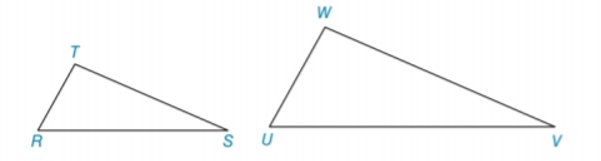Chapter 5.3, Problem 6EElementary Geometry For College St...

7th Edition
Alexander + 2 others
ISBN: 9781337614085

Solutions

Chapter
SectionElementary Geometry For College St...

7th Edition
Alexander + 2 others
ISBN: 9781337614085
Textbook Problem

In Exercises 5 to 8, name the method ( A A , S S S ~ ,   o r   S A S ~ ) that is used to show that the triangles are similar. ∠ T ≅ ∠ W and ∠ R ≅ ∠ UTo determine

To name:

The method that is used to show that the triangles are similar.

Explanation

Given:

The triangles,

TW and RU

Approach:

Two polygons are similar if and only if two conditions are satisfied.

1. All pairs of corresponding angles are congruent.

2. All pairs of corresponding sides are proportional.

Definitions:

AA:

If the two angles of one triangle are congruent to the two angles of another triangle, then the triangles are similar.

SAS:

If an angle of one triangle is congruent to an angle of a second triangle and the pairs of sides including the angles are proportional (in length), then the triangles are similar

Still sussing out bartleby?

Check out a sample textbook solution.

See a sample solution

The Solution to Your Study Problems

Bartleby provides explanations to thousands of textbook problems written by our experts, many with advanced degrees!

Get Started

Given that the point P(3, 5) lies on the line kx + 3y + 9 = 0, find k.

Applied Calculus for the Managerial, Life, and Social Sciences: A Brief Approach

Find f. f(t) = 4/(1 + t2), f(1) = 0

Single Variable Calculus: Early Transcendentals, Volume I

Evaluate the integral, if it exists. 01ex1+e2xdx

Single Variable Calculus: Early Transcendentals

Maximize Subject to P=3x4y x+y45x2y10x0,y0

Finite Mathematics for the Managerial, Life, and Social Sciences

i=1n(4i2+i)= a) 4i=1n(i2+i) b) 4i=1ni2+i=1ni c) i=1n5i2 d) 4i=1ni2+4i=1ni

Study Guide for Stewart's Single Variable Calculus: Early Transcendentals, 8th